# Ideas about electricityElectronics guide > The very first steps > Ideas about electricity

Electricity is a funny thing. Even though we know how to use it, how to make it do work for us, to amplify, to switch, to control, to create light or heat (you’ll find out about all of these aspects of electricity over the coming chapters) we can still only guess at what it is. It’s actually impossible to see electricity: we only see what it does! Sure, everyone knows that electricity is a flow of electrons, but what are electrons? Have you ever seen one? Do you know what they look like?

The truth of the matter is that we can only hypothesise about electricity. Fortunately, the hypothesis can be seen to stand in all of the aspects of electricity and electronics we are likely to look at, so to all intents and purposes the hypothesis we have is absolute. This means we can build up ideas about electricity and be fairly sure they are correct.

Right then, let’s move on to the first idea: that electricity is a flow of electrons. To put it another way, any flow of electrons is electricity. If we can measure the electricity, we must therefore be able to say how many electrons were in the flow. Think of an analogy, say, the flow of water through a pipe (Figure 1.1). The water has an evenly distributed number of foreign bodies in it. Let’s say there are ten foreign bodies (all right then, ten specks of dust) in every cm3 of water.Figure 1.1 Water flowing in a pipe is like electricity in a wire

Now, if 1 litre of water pours out of the end of the pipe into the bucket shown in Figure 1.1, we can calculate the number of specks of dust which have flowed through the pipe. There’s, as near as dammit, 1000 cm3 of water in a litre, so: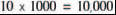water-borne specks of dust must have flowed through the pipe.

Alternatively, by knowing the number of specks of dust which have flowed through the pipe, we can calculate the volume of water. If, for example, 25,000 specks of dust have flowed, then 2.5 litres of water will be in the bucket.

Charge

It’s the same with electricity, except that we measure an amount of electricity not as a volume in litres, but as a charge in coulombs (pronounced koo-looms). The foreign bodies which make up the charge are, of course, electrons.

There’s a definite relationship between electrons and charge: in fact, there are about 6,250,000,000,000,000,000 electrons in one coulomb. But don’t worry, it’s not a number you have to remember — you don’t even have to think about electrons and coulombs because the concept of electricity, as far as we’re concerned, is not about electron flow, or volumes of electrons, but about flow rate and flow pressure. And as you’ll now see, electricity flow rate and pressure are given their own names which — thankfully — don’t even refer to electrons or coulombs.

Going back to the water and pipe analogy, flow rate would be measured as a volume of water which flowed through the pipe during a defined period of time, say 10 litres in one minute, 1,000 litres in one hour or one litre in one second.

With electricity, flow rate is measured in a similar way, as a volume which flows past a point, during a defined period of time, except that volume is, of course, in coulombs. So, we could say that a flow rate of electricity is 10 coulombs in one minute, 1,000 coulombs in one hour or one coulomb in one second.

We could say that, but we don’t! Instead, in electricity, flow rate is called current (and given the symbol I, when drawn in a diagram).

Electric current is measured in amperes (shortened to amps, or even further shortened to the unit: A), where one amp is defined as a quantity of one coulomb passing a point in one second.

Instead of saying 10 coulombs in one minute we would therefore say: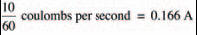Similarly, instead of a flow rate of 1,000 coulombs in one hour, we say: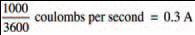The other important thing we need to know about electricity is flow pressure. Back to our analogy with water and pipe, Figure 1.2 shows a header tank of water at a height, h, above the pipe. Water pressure is often classed as a head of water, where the height, h, in metres, is the head. The effect of gravity pushes down the water in the header tank, forming a flow pressure, forcing the water out of the pipe. It’s the energy contained in the water in the header tank due to its higher position — its potential energy — which defines the water pressure.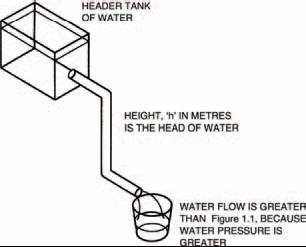Figure 1.2 A header tank’s potential energy forces the water with a higher pressure

With electricity the flow pressure is defined by the difference in numbers of electrons between two points. We say that this is a potential difference, partly because the difference depends on the positions of the points and how many electrons potentially exist. Another reason for the name potential difference comes from the early days in the pioneering of electricity, when the scientists of the day were making the first batteries. Figure 1.3 shows the basic operating principle of a battery, which simply generates electrons at one terminal and takes in electrons at the other terminal. Figure 1.3 also shows how the electrons from the battery flow around the circuit, lighting the bulb on their way round.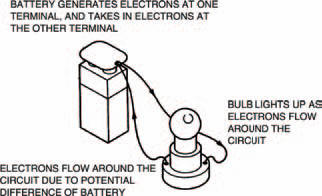Figure 1.3 A battery forces electrons around a circuit, only when the circuit is complete. If it’s not connected (see Figure 1.4 over), no electrons flow — but it still has the potential to make them flow

Under the conditions of Figure 1.4 (over), on the other hand, nothing actually happens. This is because the two terminals aren’t joined and so electrons can’t flow. (If you think about it, they are joined by air, but air is an example of a material which doesn’t allow electrons to flow through it under normal conditions. Air is an insulator or a non-conductor.) Nevertheless the battery has the potential to light the bulb and so the difference in numbers of electrons between two points (terminals in the case of a battery) is known as the potential difference. A more usual name for potential difference, though, is voltage, shortened to volts, or even the symbol V. Individual cells are rated in volts and so a cell having a voltage of 3 V has a greater potential difference than a cell having a voltage of 2 V. The higher the voltage, the harder a cell can force electrons around a circuit. Voltage is simply a way of expressing electrical pushing power.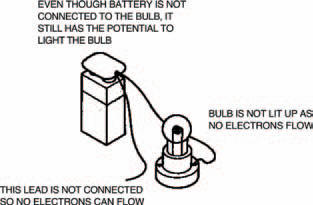Figure 1.4 Even when the battery is disconnected and electrons do not flow, the battery still has a potential difference